Exam 2,  Essentials of Physics—PHYS 101—  May 23, 2007

This exam consists of 16 multiple choice questions (worth 2 points each).  Please put your name and student ID on the Scantron form and indicate your answers to the multiple choice questions on it.  Please fill in the circles below your student ID on the scantron as well as writing it.  Important formulae and conversion factors can be found on the last page.

_33_1) We humans and everything else in the world travel in a circular path as the Earth rotates daily about its polar axis.  This means that:

A)  There is a centripetal force acting on us, which is caused by the rotating Earth.

B)  There is a centrifugal force acting on us, which is caused by gravity.

C)  There is a centrifugal force acting on us, causing us to turn in a circle.

D)  There are both centrifugal and centripetal forces acting on us, which just cancel each other out.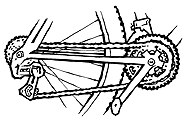E)  There is a centripetal force acting on us, which is caused by gravity.

73_2)   When you shift the chain your bicycle to a larger rear gear, while pushing the same on the pedals, you exert:

A)  less torque on the gear and, thus, your rear tire.

B)  the same torque on the gear and, thus, your rear tire.

C)  more torque on the gear and, thus, your rear tire.

19_3)   You are designing a car for the Soap Box Derby, where scale model cars race down hills pulled only by gravity.  Which type of front wheels will give you the best chance of winning the race?

A)  Light disk-like wheels where the weight is distributed from the center to the rim.

B)  Heavy disk-like wheels where the weight is distributed from the center to the rim.

C)  Light hoop-like wheels where all the weight is at the rim.

D)  Heavy hoop-like wheels where all the weight is at the rim.

61_4)   Planets would crash into the sun if it weren’t for:

A)  their tangential velocities.

B)  their vast distance from the sun.

C)  the fact that they are beyond the main gravitation of the sun.

D)  the inverse-square law.

E)  their relatively small masses.

72_5)   In the illustration, below, with the moon at the position shown along the dashed-line orbit (below) what is the phase of the moon (below, right)?                                                                    A;   B;   C;   D;     or E (none of these)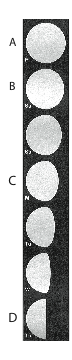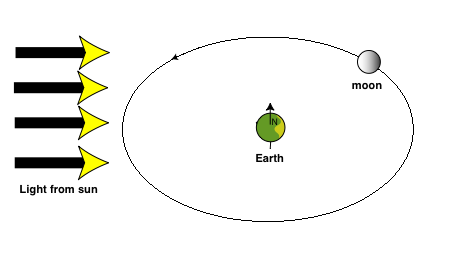94_6)   A woman on the surface of the Earth has a mass of 50 kilograms and a weight of 490 newtons.  If the woman were floating freely inside a space habitat far from Earth, she would have:

A)  less weight and more mass.

B)  less weight and the same mass.

C)  less weight and less mass.

D)  more weight and less mass.

E)  the same weight and less mass.

78_7)   In the heliocentric (sun-centered) model for the solar system, the Earth has seasons because:

A)  the Earth’s axis is tilted relative to the plane of the Earth-moon system.

B)  the Earth’s axis is tilted relative to the plane of the Earth-Sun system.

C)  the Sun projects more intense light in certain directions.

D)  the Sun moves closer and further away as it orbits the Earth.

E)  the Earth moves closer and further away from the Sun as it orbits the Sun.

83_8)   A “weightless” astronaut in an orbiting shuttle is:

A)  pulled only by gravitation to the shuttle which cancels Earth’s gravitational pull.

B)  like the shuttle, pulled by Earth’s gravitation and falling towards Earth.

C)  in a situation where their centripetal force is just canceled by their gravitational force.

D)  shielded from Earth’s gravitational field.

E)  beyond the pull of gravity.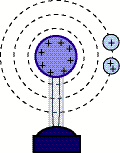33_9)   Two charged bodies are brought to the same distance away from a charged Van de Graaf generator.  Both are identical except that one has twice the charge as the other.  What is true about the charged bodies and their relationship to the Van de Graaf?

A) The body with more charge has greater voltage, but the same potential energy as the other.

B) The body with more charge has greater potential energy but the same voltage as the other.

C) Both bodies have the same potential energy, and the same voltage.

D) Both bodies have different potential energies and different voltages.

36_10) When two identical household lamps are connected in parallel to the power supply, the electrical current that the power supply provides is:

A) four times the current for just one lamp       D) half the current for just one lamp.

B) two times the current for just one lamp        E) one-quarter the current for just one lamp.

C) the same as the current for just one lamp.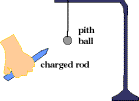69_11) In class we moved a charged rod near an uncharged pith ball.  What happened at first, and why?

A)  the unbalanced charges on the rod attracted the opposite charges on the ball, causing the ball to move away from the rod.

B)  the unbalanced charges on the rod attracted the opposite charges on the ball, causing the ball to move towards the rod.

C)  the unbalanced charges on the rod charged the ball, causing the ball to move away from the rod.

D)  the unbalanced charges on the rod charged the ball, causing the ball to move towards the rod.

E)  protons on the ball moved towards electrons on the rod, causing the ball to move towards the rod.

53_12) Which circuit below correctly depicts measurement of the current and voltage for bulb 2 using a voltmeter (circled V, below) and an ammeter (circled A, below)?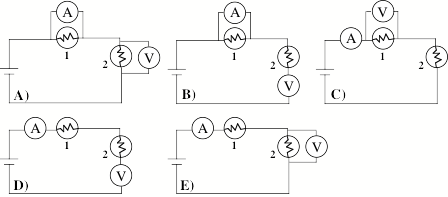A.  A)              B.  B)              C.  C)              D.  D)              E. E)

86_13) When we say an appliance “uses up electricity,” we really are saying that:

A) current disappears.

B) electron kinetic energy is changed into some other kind of energy.

C) electric charges go away.

D) electrons are taken out of the circuit and put somewhere else.

E) the power supply voltage is substantially reduced.

72_14) Choose the best explanation as to why Bulb A brightens when the switch is closed.

A)  the battery gets stronger when another obstacle is added to the circuit.

B)  the current increases when another obstacle is added to the circuit.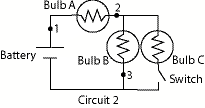C)  Bulb B gets brighter, so Bulb A also must get brighter.

D)  Adding Bulb C adds another path to the circuit, so current through point 1 must increase.

E)  Adding Bulb C adds another path to the circuit, so current through point 3 must increase.

86_15) An object that is electrically charged has:

A)  the same number of neutrons and protons.

C)  the same number of electrons and protons.

83_16) A main difference between gravitational and electric forces is that electrical forces:

A)  act over shorter distances but stop working over long distances.

B)  are weaker.

C)  attract.

D)  repel or attract.

E)  obey the inverse-square law.

Formulae:

Work:             Work = Force x displacment            {N x m}  (force and disp. in same direction)

Gravitational Potential Energy (GPE):

GPE = m g h {J}       (g=acceleration of gravity, h=height)

Kinetic Energy (KE):            KE = 1/2 m v2 {J}      (v=velocity)

Power: (P):                             P = work / time (W)

Angular Momentum:           Angular momentum = m v r (kg m2 / s)

Universal Law of Gravity:

F = G m1 m2 / d2  (N)             where G is a constant, m1 and m2 are masses of two                                                                          objects, and d is the distance between the objects.

Coulomb’s Law:      F = k q1 q2 / d2,  where F is force, k is a constant, q’s are charges and d is distance between charges.

Ohm’s Law:             V = I R,   where V is voltage (electric potential difference), I is current and R is resistance.

Electric Power:         P = I V,  where P is power.

Electric Potential:     V = EPE / charge,   (EPE is Electric Potential Energy)